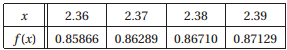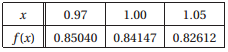Create an Account

Home / Questions / 1 Use finite difference approximations of Oh2 to compute f236 and f 2 36 from the data ...

1 Use finite difference approximations of Oh2 to compute f236 and f 2 36 from the data 2 Estimate f1 and f1 from the following data

1. Use finite difference approximations of O(h2) to compute f’(2.36) and f”(2.36) from the data2. Estimate f’(1) and f”(1) from the following data:Jul 27 2020 View more View LessSubscribe To Get Solution